Go BACK
Getting Started

# Noise

## Noise Type vs Noise Base

The only thing that changes between the images below are the Noise Type and Noise Base. All other settings are defaults

Example:

## Noise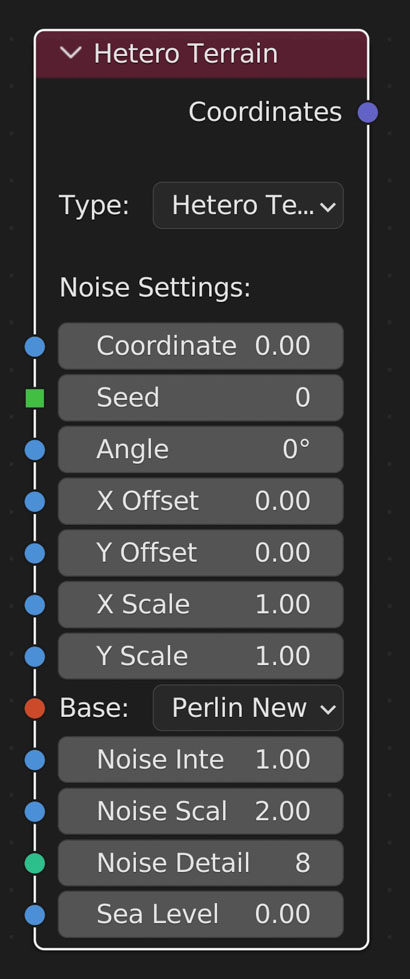Create noise of type with given base

• Type:
-
The type of noise to calculate
• Coordinates:
-
The coordinates to input into the noise function
• Seed:
-
Finds a new random offset for the noise
• Angle:
-
Rotates the noise pattern without rotating the object
• X Offset:
-
Moves the noise in the X axis
• Y Offset:
-
Moves the noise in the Y axis
• X Scale:
-
Scales the noise in the X axis
• Y Scale:
-
Scales the noise in the Y axis
• Base:
-
The type of underlying noise to use
• Noise Intensity (H):
-
Makes noise more or less pointy. Smaller values increase intensity. This is the fractal increment factor.
• Noise Scale (Lacunarity):
-
Scales noise. The gap between successive frequencies.
• Noise Detail (Octaves):
-
Level of noise detail. The number of different noise frequencies used.
• Sea Level:
-
The height of the terrain above ‘sea level’.
• Gain:
-
Scaling applied to the values.
• Amplitude Scale:
-
The amplitude scaling factor.
• Frequency Scale:
-
The frequency scaling factor
• Distance Metric: (Voronoi)
-
Distance, Distance Squared, Manhattan, Chebychev, Minkovsky, Minkovsky Half, Minkovsky Four
• Exponent: (Voronoi)
-
The exponent for the Minkowski distance metric
• Distortions: (Variable Lacunarity)
-
The amount of distortion
• Hard:
-
Changes the noise to have hard edges in certain places
• Turbulence: (Marble)
-
The amount of turbulence (randomness)Create noise of type with given base

• Type:
-
The type of noise to calculate
• Coordinates:
-
The coordinates to input into the noise function
• Seed:
-
Finds a new random offset for the noise
• Angle:
-
Rotates the noise pattern without rotating the object
• X Offset:
-
Moves the noise in the X axis
• Y Offset:
-
Moves the noise in the Y axis
• X Scale:
-
Scales the noise in the X axis
• Y Scale:
-
Scales the noise in the Y axis
• Base:
-
The type of underlying noise to use
• Noise Intensity (H):
-
Makes noise more or less pointy. Smaller values increase intensity. This is the fractal increment factor.
• Noise Scale (Lacunarity):
-
Scales noise. The gap between successive frequencies.
• Noise Detail (Octaves):
-
Level of noise detail. The number of different noise frequencies used.
• Sea Level:
-
The height of the terrain above ‘sea level’.
• Gain:
-
Scaling applied to the values.
• Amplitude Scale:
-
The amplitude scaling factor.
• Frequency Scale:
-
The frequency scaling factor
• Distance Metric: (Voronoi)
-
Distance, Distance Squared, Manhattan, Chebychev, Minkovsky, Minkovsky Half, Minkovsky Four
• Exponent: (Voronoi)
-
The exponent for the Minkowski distance metric
• Distortions: (Variable Lacunarity)
-
The amount of distortion
• Hard:
-
Changes the noise to have hard edges in certain places
• Turbulence: (Marble)
-
The amount of turbulence (randomness)

### Blender Noise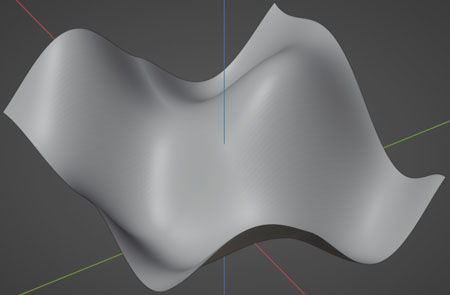### Cell Noise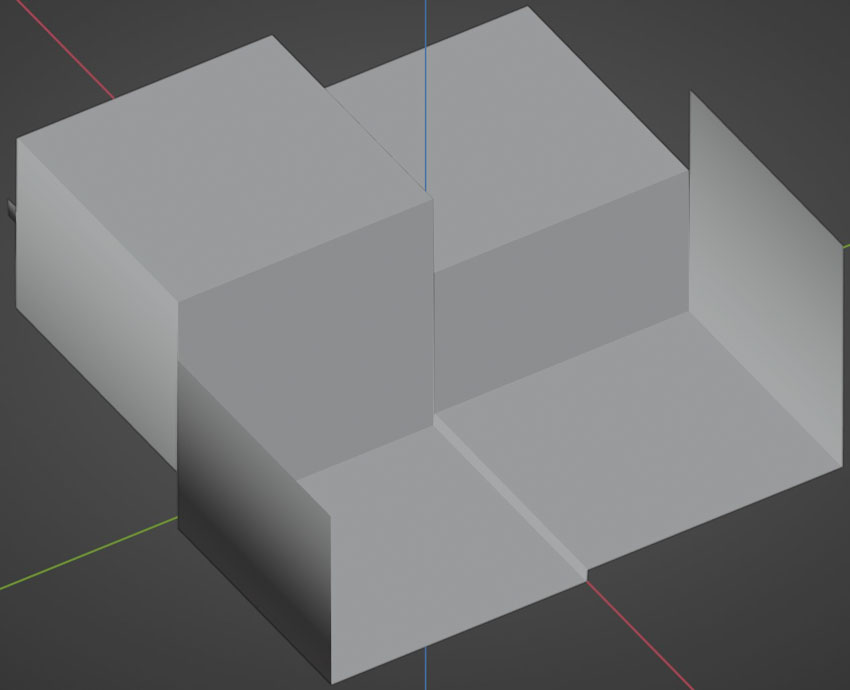### Perlin New Noise### Random Noise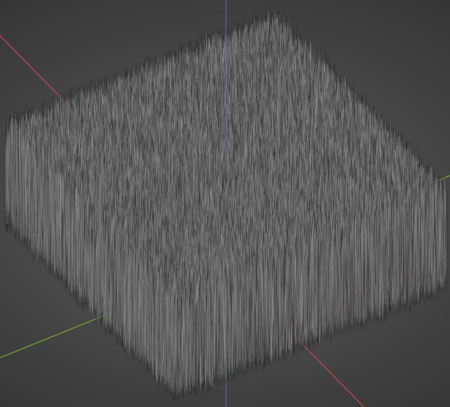### Voronoi Crackle Noise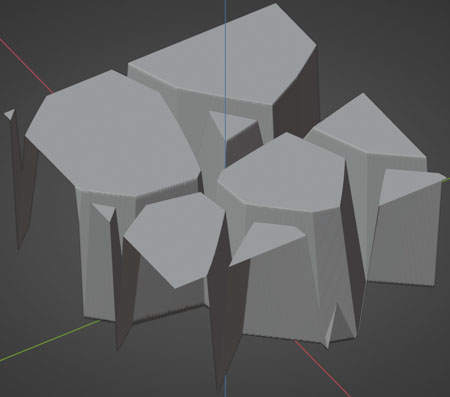### Voronoi Distance: Chebychev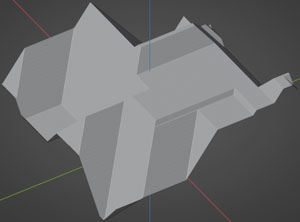### Voronoi Distance: Distance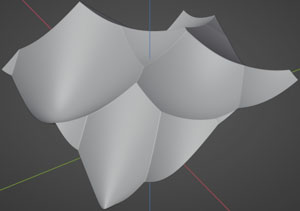### Voronoi Distance: Distance Squared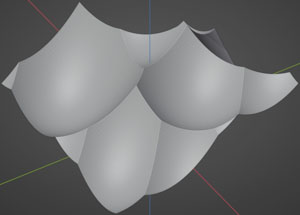### Voronoi Distance: Manhattan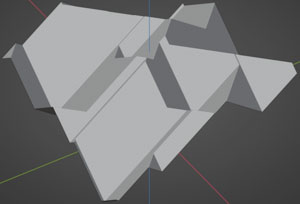### Voronoi Distance: Minkovsky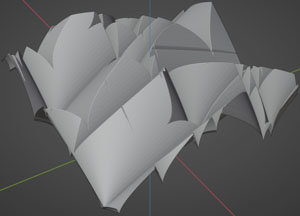### Voronoi Distance: Minkovsky Four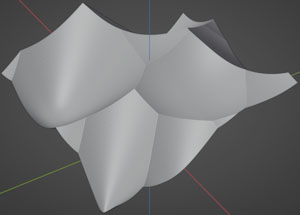### Voronoi Distance: Minkovsky Half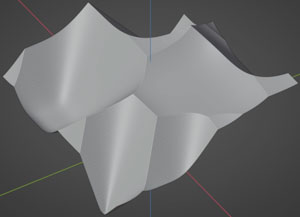### Voronoi F1 Noise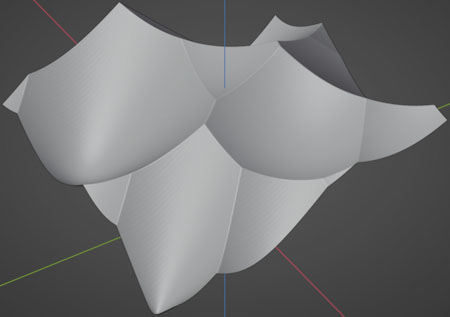### Voronoi F2 Noise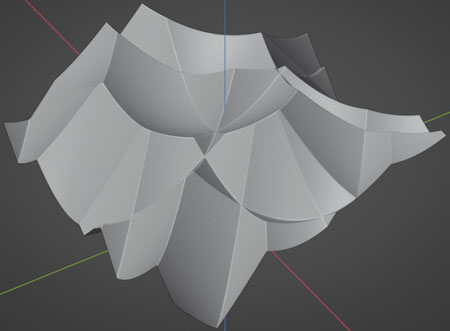### Voronoi F2F1 Noise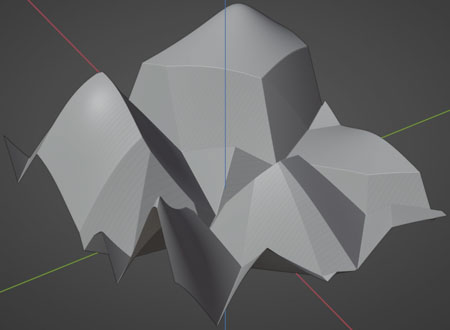### Voronoi F3 Noise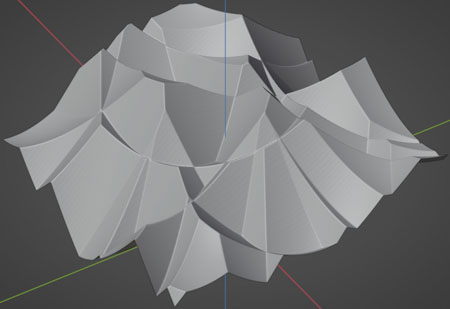### Voronoi F4 Noise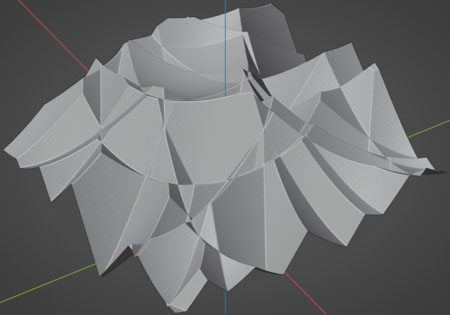Created:
Monday, August 7, 2023
updated:
Wednesday, August 9, 2023

#### True-VFX

Author |
Heather @ True-VFX